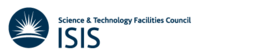SpinW is a MATLAB library that can plot and numerically simulate magnetic structures and excitations of given spin Hamiltonian using classical Monte Carlo simulation and linear spin wave theory.

# The Projects

## Features

In short SpinW can solve the following spin Hamiltonian using classical and quasi classical numerical methods:

$\mathcal{H}=\sum_{i,j}\mathbf{S}_iJ_{ij}\mathbf{S}_j + \sum_i \mathbf{S}_iA_i\mathbf{S}_i + \mathbf{B}\sum_i\mathbf{g}_i\mathbf{S}_i$

where $S_i$ are spin vector operators, $J_{ij}$ are 3x3 matrices describing pair coupling between spins, $A_{ij}$ are 3x3 anisotropy matrices, $B$ is external magnetic field and $g_i$ is the g-tensor.

### Crystal structures

• definition of crystal lattice with arbitrary unit cell, using space group or symmetry operators
• definition of non-magnetic atoms and magnetic atoms with arbitrary moment size
• publication quality plotting of crystal structures (atoms, labels, axes, surrounding polyhedron, anisotropy ellipsoids, DM vector, etc.)

### Magnetic structures

• definition of 1D, 2D and 3D magnetic structures
• representation of incommensurate structures using rotating coordinate system or complex basis vectors
• generation of magnetic structures on a magnetic supercell
• plotting of magnetic structures

### Magnetic interactions

• simple assignment of magnetic interactions to neighbouring magnetic atoms based on distance
• possible interactions: Heisenberg, Dzyaloshinskii-Moriya, anisotropic and general 3x3 exchange tensor
• arbitrary single ion anisotropy tensor (easy-plane, easy-axis, etc.)
• Zeeman energy in homogeneous magnetic field including arbitrary g-tensor
• calculation of symmetry allowed elements of the above tensors based on the crystallographic space group

### Simulation of magnetic structures

• classical energy minimization assuming single-k magnetic structure for fast and simple solution for ground state magnetic structure
• simulated annealing using the Metropolis algorithm on an arbitrary large magnetic supercell
• calculating properties in thermodynamical equilibrium (heat capacity, magnetic susceptibility, etc.)
• magnetic structure factor calculation using FFT
• simulation of magnetic neutron diffraction and diffuse scattering
• Simulation of magnetic excitations in general commensurate and incommensurate magnetic structures using linear spin-wave theory
• calculation of spin wave dispersion, spin-spin correlation functions
• calculation of neutron scattering cross section for unpolarized neutrons including the magnetic form factor
• calculation of polarized neutron scattering cross sections
• possible to include different moment sizes for different magnetic atoms
• calculation of powder averaged spin wave spectrum

### Plotting of spin wave spectrum

• plotting of dispersions and correlation functions
• calculation and plotting of the convoluted spectra for direct comparison with inelastic neutron scattering
• full integration into Horace for plotting and comparison with time of flight neutron data, see http://horace.isis.rl.ac.uk

### Fitting spin wave spectra

• possible to fit any parameter in the Hamiltonian
• robust fitting, even when the number of simulated spin wave modes differs from the measured number of modes

# Our Partners